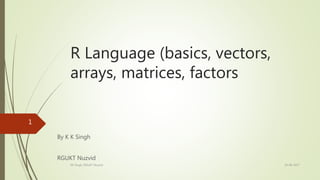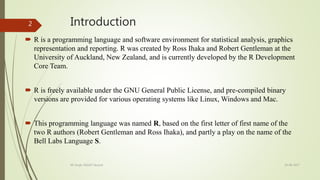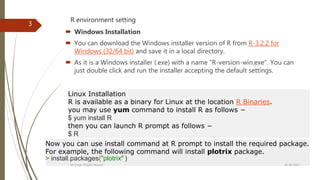Se está descargando tu SlideShare. ×

# 2. R-basics, Vectors, Arrays, Matrices, Factors

Anuncio
Anuncio
Anuncio
Anuncio
Anuncio
Anuncio
Anuncio
Anuncio
Anuncio
Anuncio
AnuncioCargando en…3
×

1 de 21 Anuncio

# 2. R-basics, Vectors, Arrays, Matrices, Factors

It covers- Introduction to R language, Creating, Exploring data with Various Data Structures e.g. Vector, Array, Matrices, and Factors. Using Methods with examples.

It covers- Introduction to R language, Creating, Exploring data with Various Data Structures e.g. Vector, Array, Matrices, and Factors. Using Methods with examples.

Anuncio
Anuncio

### 2. R-basics, Vectors, Arrays, Matrices, Factors

1. 1. R Language (basics, vectors, arrays, matrices, factors By K K Singh RGUKT Nuzvid 19-08-2017KK Singh, RGUKT Nuzvid 1
2. 2. Introduction  R is a programming language and software environment for statistical analysis, graphics representation and reporting. R was created by Ross Ihaka and Robert Gentleman at the University of Auckland, New Zealand, and is currently developed by the R Development Core Team.  R is freely available under the GNU General Public License, and pre-compiled binary versions are provided for various operating systems like Linux, Windows and Mac.  This programming language was named R, based on the first letter of first name of the two R authors (Robert Gentleman and Ross Ihaka), and partly a play on the name of the Bell Labs Language S. 19-08-2017KK Singh, RGUKT Nuzvid 2
3. 3. R environment setting  Windows Installation  You can download the Windows installer version of R from R-3.2.2 for Windows (32/64 bit) and save it in a local directory.  As it is a Windows installer (.exe) with a name "R-version-win.exe". You can just double click and run the installer accepting the default settings. Linux Installation R is available as a binary for Linux at the location R Binaries. you may use yum command to install R as follows − \$ yum install R then you can launch R prompt as follows − \$ R Now you can use install command at R prompt to install the required package. For example, the following command will install plotrix package. > install.packages("plotrix" ) 19-08-2017KK Singh, RGUKT Nuzvid 3
4. 4. Basic Sentence Start your R command prompt by typing the following command (in Linux) − \$ R OR double click on installed .exe file ( in windows) This will launch R interpreter and you will get a prompt > where you can start typing your program as follows −  myString <- "Hello, World!"  > print ( myString)  "Hello, World!" R Script File Usually, you will do your programming by writing your programs in script files and execute those scripts with the help of R interpreter called Rscript. Ex: # My first program in R Programming myString <- "Hello, World!“ print ( myString) Save it as test.R and execute it at R command prompt. > source(“test.R”)  "Hello, World!" 19-08-2017KK Singh, RGUKT Nuzvid 4
5. 5. Some basic useful command  >help(word) # get the description of the word  >?word # get the description  >getwd() # get the working directory  >setwd(“C:/kk”) # set the working directory  >q() # quit  >source(“filename.R”) #execute Rscript  ……………………………………………………………..  >sink(“filename.txt”) # direct output into filenale.txt  >source(“file.R”)  >sink() #exit from sink mode 19-08-2017KK Singh, RGUKT Nuzvid 5
6. 6. R –Data Types  In contrast to other programming languages like C and java in R, the variables are not declared as some data type.  The variables are assigned with R-Objects and the data type of the R- object becomes the data type of the variable.  Vectors  Arrays  Matrices  Lists  Factors  Data Frames 19-08-2017KK Singh, RGUKT Nuzvid 6
7. 7. Vector-Data Types Data Type Example Verify Logical TRUE, FALSE v <- TRUE print(class(v))  "logical" Numeric 12.3, 5, 999 v <- 23.5 print(class(v))  "numeric" Integer 2L, 34L, 0L v <- 2L print(class(v))  "integer" Complex 3 + 2i v <- 2+5i print(class(v))  "complex" Character 'a' , '"good", "TRUE", '23.4' v <- "TRUE" print(class(v))  "character" 19-08-2017KK Singh, RGUKT Nuzvid 7
8. 8. Vector (Cont..) To create vector with more than one element, use c() function which combines elements into a vector. # Create a vector. apple <- c('red','green',"yellow") print(apple) # Get the class of the vector. print(class(apple)) ……………………………………………………………………….. The non-character values are coerced to character type if one of the elements is a character. s <- c('apple','red',5,TRUE) print(s) it produces the following result −  "apple" "red" "5" "TRUE" 19-08-2017KK Singh, RGUKT Nuzvid 8
9. 9. Vector (Cont..) Multiple Elements Vector Using colon operator with numeric data # Creating a sequence from 5 to 13. v <- 5:13 print(v) …………………………………………………………………………………………… v <- 6.6:12.6 # Creating a sequence from 6.6 to 12.6 print(v) ……………………………………………………………………………………………… # If the final element not belong to the sequence, it is discarded. v <- 3.8:11.4 print(v) ……………………………………………………………………… Using sequence (Seq.) operator # Create vector with elements from 5 to 9 incrementing by 0.4. print(seq(5, 9, by = 0.4)) ………………………………………………………………………. # empty vector >x<-numeric() >x<-5 >x 19-08-2017KK Singh, RGUKT Nuzvid 9
10. 10. Accessing vector elements Accessing Vector Elements Elements of a Vector are accessed using indexing. The [ ] brackets are used for indexing. Indexing starts with position 1. Giving a negative value in the index drops that element from result. TRUE, FALSE or 0 and 1 can also be used for indexing. # Accessing vector elements using position. t <- c("Sun","Mon","Tue","Wed","Thurs","Fri","Sat") u <- t[c(2,3,6)] print(u) ……………………………………………………………………………………………………. v <- t[c(TRUE,FALSE,FALSE,FALSE,FALSE,TRUE,FALSE)] print(v) …………………………………………………………………………………………………………… …… x <- t[c(-2,-5)] # print t excluding 2nd & 5th index value print(x) 19-08-2017KK Singh, RGUKT Nuzvid 10
11. 11. Vector Manipulation Two vectors of same length can be added, subtracted, multiplied or divided giving the result as a vector output. # Create two vectors. v1 <- c(3,8,4,5,0,11) v2 <- c(4,11,0,8,1,2) add.result <- v1+v2 print(add.result) …………………………………………………………………………… sub.result <- v1-v2 print(sub.result) ………………………………………………………………………………………………… multi.result <- v1*v2 print(multi.result) …………………………………………………………………………………………. divi.result <- v1/v2 print(divi.result) ………………………………………………………………………………………………………….19-08-2017KK Singh, RGUKT Nuzvid 11 What is output
12. 12. Vector Element Sorting Elements in a vector can be sorted using the sort() function. v <- c(3,8,4,5,0,11, -9, 304) # Sort the elements of the vector. sort.result <- sort(v) print(sort.result) # Sort the elements in the reverse order. revsort.result <- sort(v, decreasing = TRUE) print(revsort.result) 19-08-2017KK Singh, RGUKT Nuzvid 13
13. 13. Arrays  Arrays are the R data objects which can store data in more than two dimensions.  For example − If we create an array of dimension (2, 3, 4) then it creates 4 rectangular matrices each with 2 rows and 3 columns.  Arrays can store only one data type.  An array is created using the array() function.  Example creates an array of two 3x3 matrices each with 3 rows and 3 columns. vector1 <- c(5,9,3) vector2 <- c(10,11,12,13,14,15) # Take these vectors as input to the array. result <- array(c(vector1,vector2),dim = c(3,3,1)) print(result) 19-08-2017KK Singh, RGUKT Nuzvid 14
14. 14. Naming Columns and Rows We can give names to the rows, columns and matrices in the array by using the dimnames parameter. # Create two vectors of different lengths. vector1 <- c(5,9,3) vector2 <- c(10,11,12,13,14,15) column.names <- c("COL1","COL2","COL3") row.names <- c("ROW1","ROW2","ROW3") matrix.names <- c("Matrix1","Matrix2") # Take these vectors as input to the array. result <- array(c(vector1,vector2),dim = c(3,3,2),dimnames = list(row.names,column.names, m print(result) 19-08-2017KK Singh, RGUKT Nuzvid 16
15. 15. Accessing Array Elements# see previous example print(result[3,,2]) # Print the third row of the second matrix of the array. print(result[1,3,1]) # Print the element in the 1st row and 3rd column of the 1st matrix. print(result[,,2]) # Print the 2nd Matrix. It produces the following result − COL1 COL2 COL3 3 12 15  13 COL1 COL2 COL3 ROW1 5 10 13 ROW2 9 11 14 ROW3 3 12 15 Q. Access 2nd & 4th column of the result. 19-08-2017KK Singh, RGUKT Nuzvid 18
16. 16. Manipulating Array Elements As array is made up matrices in multiple dimensions, the operations on elements of array are carried out by accessing elements of the matrices. # Create two vectors of different lengths. vector1 <- c(5,9,3) vector2 <- c(10,11,12,13,14,15) array1 <- array(c(vector1,vector2),dim = c(3,3,2)) vector3 <- c(9,1,0) vector4 <- c(6,0,11,3,14,1,2,6,9) array2 <- array(c(vector1,vector2),dim = c(3,3,2)) matrix1 <- array1[,,2] matrix2 <- array2[,,2] result <- matrix1+matrix2 print(result) 19-08-2017KK Singh, RGUKT Nuzvid 19
17. 17. R-Matrix > Matrices are the R objects, which is two dimensional array. > They contain elements of the same atomic types > Matrix is created using the matrix() function. Syntax matrix(data, nrow, ncol, byrow, dimnames) Example M <- matrix(c(3:14), nrow = 4, byrow = TRUE) print(M) N <- matrix(c(3:14), nrow = 4, byrow = FALSE) # Elements are arranged sequentially by column. print(N) # Define the column and row names. rownames = c("row1", "row2", "row3", "row4") colnames = c("col1", "col2", "col3") P <- matrix(c(3:14), nrow = 4, byrow = TRUE, dimnames = list(rownames, colnames)) print(P) 19-08-2017KK Singh, RGUKT Nuzvid 21
18. 18. Accessing Elements of a Matrix Elements of a matrix can be accessed by using the column and row index of the element. rownames = c("row1", "row2", "row3", "row4") colnames = c("col1", "col2", "col3") P <- matrix(c(3:14), nrow = 4, byrow = TRUE, dimnames = list(rownames, colnames)) # Create the matrix print(P[1,3]) # Access the element at 3rd column and 1st row. print(P[4,2]) # Access the element at 2nd column and 4th row. print(P[2,]) # Access only the 2nd row. print(P[,3]) # Access only the 3rd column. It produces the following result −  5  13 col1 col2 col3 6 7 8 row1 row2 row3 row4 5 8 11 14 Q. A matrix has 100 columns, access all even index column. 19-08-2017KK Singh, RGUKT Nuzvid 23
19. 19. 19-08-2017KK Singh, RGUKT Nuzvid 24 Factorsare the data objects which are used to categorize the data and store it as levels. They can store both strings and integers. They are useful in the columns which have a limited number of unique values. Like "Male, "Female" and True, False etc. They are useful in data analysis for statistical modeling. Factors are created using the factor () function by taking a vector as input. Example # Create a vector as input. data <- c("East","West","East","North","North","East","West","West","West","East","North") print(data) print(is.factor(data)) # Apply the factor function. factor_data <- as.factor(data) print(factor_data) print(is.factor(factor_data)) It produces the following result −  "East" "West" "East" "North" "North" "East" "West" "West" "West" "East" "North"  FALSE  East West East North North East West West West East North Levels: East North West  TRUE
20. 20. 19-08-2017KK Singh, RGUKT Nuzvid 25 Factors in Data Frame On creating any data frame with a column of text data, R treats the text column as categorical data and creates factors on it. # Create the vectors for data frame. height <- c(132,151,162,139,166,147,122) weight <- c(48,49,66,53,67,52,40) gender <- c("male","male","female","female","male","female","male") input_data <- data.frame(height,weight,gender) print(input_data) # Test if the gender column is a factor. print(as.factor(input_data\$gender)) # Print the gender column so see the levels. print(input_data\$gender)
21. 21. 19-08-2017KK Singh, RGUKT Nuzvid 27